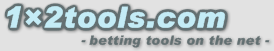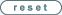BET CALCULATOR
1. PREFERENCES
Event:
 1X2 Totals
Number of outcomes:
 2 3 4 5 6 7
Commission for price limits (%):
Stake divider: Bankroll:
2. PROBABILITIES
3. BET DATA
bet limit com price bookie price
EV
optimal
stake
bankroll
growth rate4. RESULTA Kelly calculator and an asian handicaps calculator in one. This is a beta-version so be careful!

Instructions

1. Preferences:
-Choose the event type (sides or totals). Please allow to your browser a few seconds to re-render the tool layout after changing this option.
-Select the number of outcomes. The more outcomes, the more betting options you have, but also, the more probabilities you will need. Here again, please allow to your browser a few seconds to re-render the tool layout after changing this option.
-Enter other preferences also now.

2. Probabilities:
-Enter probabilities in percentage format. The sum of the probabilities must be 100%.
-Press the "Calculate" button. The tool will calculate price limits. These limits will help you to find value bets.

3. Bet Data:
-Hunt the markets for value odds and enter them into the calculator. Press the "Calculate" button. The tool will calculate the most profitable single bet.

-"DO" stands for "draw option".
-"com" stands for "commission".
-"EV" stands for "estimated value".
-Please allow pop-up -messages for error reporting.

If you want to make "combo bets" - or in other words, combine asian handicaps and totals for example - you should try Advanced Bet Calculator (below).

 ARBITRAGE CALCULATOR
 Commis-sion % Price (decimal) Bookie (optional) Arbitrage limit Zero profits Key Stake Stake Profit Return %: Stakes in total:This tool is for arbitrage betting but it can also be used to other tasks, for example one can calculate the competitiveness of the market easily.

Instructions:
1. Enter the prices to the "Price" column (and don't forget the commissions).
2. On the bottom left corner is the "Return Percentage" field. If the value of the field is 100% or more, there is an arbitrage situation.
3. In the "Arbitrage limit" column you can see what should be the price so that the return percentage of the market would be 100%. If an arbitrage situation is unreachable there will be reading "arb n.a" (arbitrage not available) which means that there will be no arbitrage situation no matter what the price would be.
4. In the "Zero Profits" column you can choose that you will get more profit from certain match results and no profit from others. Just click the checkbox.
5. Choose the key stake in the "Key Stake" column. The key stake will define stakes for the other bets.
6. Enter the key stake. Stakes for the other bets will be calculated automatically.
 Home Team: Visiting Team:Stake Divider: Maximum investment(0-1000% from the bankroll): Bankroll (€): Tip: If the "Stake Divider" is more than one, the stakes will be divided by that number. The "Maximum Investment" defines how much at most you are willing to invest to the match.
Total goals:

Sum:Tip: The probabilities must be set in a percen­tage format and the sum of the probabi­lities must be exactly 100%. Please check the following examples to find out the logic how the probabilities should be set: example 1, example 2, example 3. Finally by pressing the "Update" button the tool will calculate the limits and you can move to section 3.Tip: Calculating may take 1 to 30 seconds.
 Optimized wagering solution will appear here.
Bet type Limit Price Bookie Pend-
ing wager price
Pend-
ing wager stake
Recom-
mended stake
Asian Handicaps:

Draw Options:

Totals:Tip: Search the markets for prices and add them into the "Price" column. You can also write the bookie to the "Bookie" column. If you already have placed wagers to the event you should add them into the "Pending wager price" and the "Pending wager stake" columns. Please note that the maximum amount of data rows has been limited to 8. When you have entered all the required data you can press the "optimize" button in section 4.

# KELLY CALCULATOR

 price (decimal): commission (%): probability (%): stake divider: bankroll:optimal stake: bankroll growth rate:

# AHC - KELLY CALCULATOR

 price (decimal): commission (%): P(win) (%): P(½win, ½push) (%): P(push) (%): P(½push, ½loss) (%): stake divider: bankroll:optimal stake: bankroll growth rate:

# PRICE INVERSER

 price (decimal):inversed price:

# COMMISSION ELIMINATOR

 price (decimal): commission (%):net price:

 net price (decimal): commission (%):price with commission:

# LAY CALCULATOR

 price offered: risk:backer's stake:

# PRICE CONVERTER

 American (+000) Decimal (0.00) Fraction (5/3)# COVER BETS

 main bet: price (decimal): stake: cover bet: price (decimal): cover %:stake for the cover bet: result when the main bet hits: result when the cover bet hits: result when none of the bets hits:

# WAGER COMBINER

 wager #1: price: stake: wager #2: price: stake:combined price: combined stake:

# SINGLE BET PROFITS

 price (decimal): stake:straight win: half win + half push: half push + half loss:

# CALCULATOR

formula:result:

# TARGET PROFITS

 price (decimal): commission (%): profit wanted:stake needed:

# ODDS COMPILER

 prices to probabilities probabilities to prices scale to 100% return
price
(decimal)
probability
(%)
=
=
=
=
=
=
=
sum of the probabilities:
price return %:# TIPSTERS' AVERAGES

 probability 1 (%): probability 2 (%): tipster 2 weight %:linearly weighted probability: